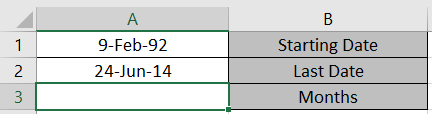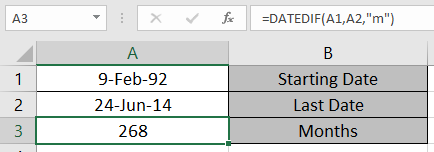# How to Calculate Months Between dates in Excel

In this article, we will learn how to calculate the number of months elapsed between dates using DATEDIF function in Excel.

DATEDIF function returns the number of years, months and days between the given two dates in Excel.
Syntax:

=DATEDIF(start_date, end_date, unit)

Unit:
“y” denotes Years between dates
“m” denotes between dates
“d” denotes days between dates
"md" denotes days between dates, ignoring months and years
"ym" denotes months between dates, ignoring days and years
"yd" denotes days between dates, ignoring years

Let’s understand this using it in an exampleUse the formula

=DATEDIF(A1,A2,"m")As you can see the function returns the number of months between two given dates.

If you wish to find days or years between two given dates.

Hope you understood How to use DATEDIF function to calculate the months between two dates. Explore more articles on Excel Date & Time Functions here. Please state your queries in the comment box below.

Popular Articles:

50 Excel Shortcuts to Increase Your Productivity

How to use the VLOOKUP Function in Excel

How to use the COUNTIF function in Excel

How to use the SUMIF Function in Excel

Terms and Conditions of use

The applications/code on this site are distributed as is and without warranties or liability. In no event shall the owner of the copyrights, or the authors of the applications/code be liable for any loss of profit, any problems or any damage resulting from the use or evaluation of the applications/code.